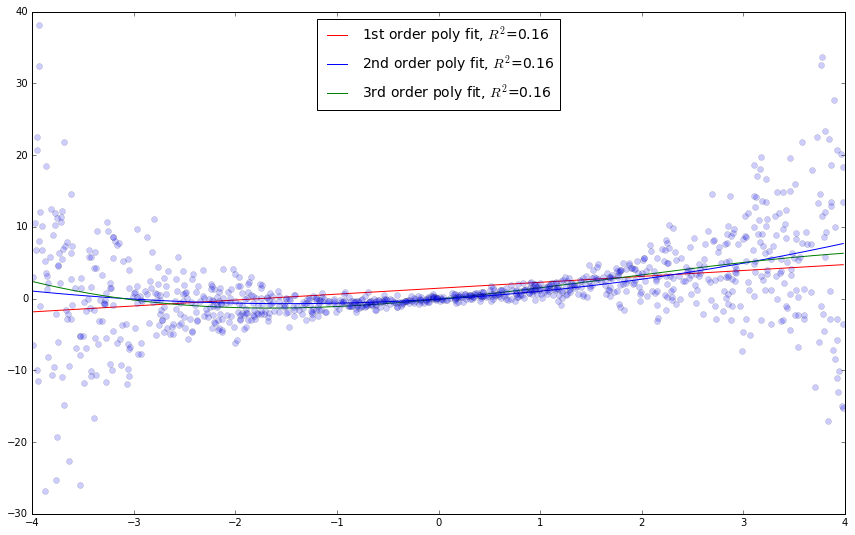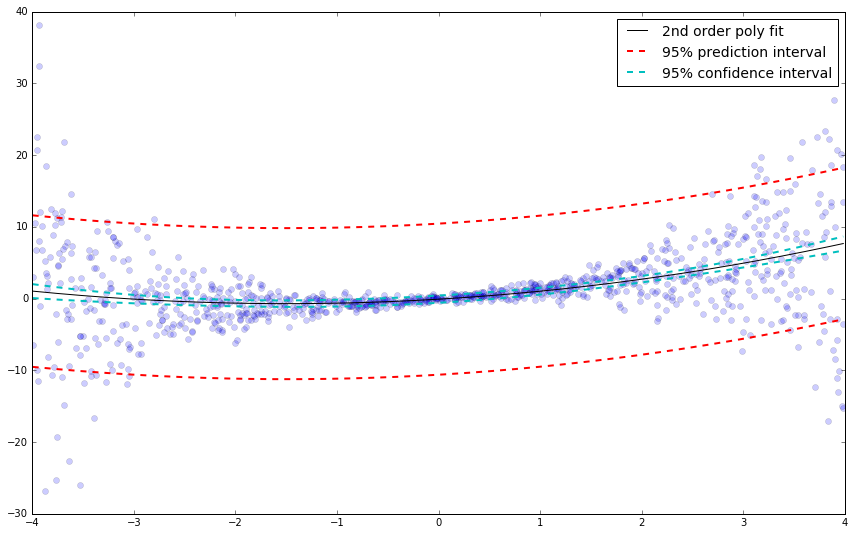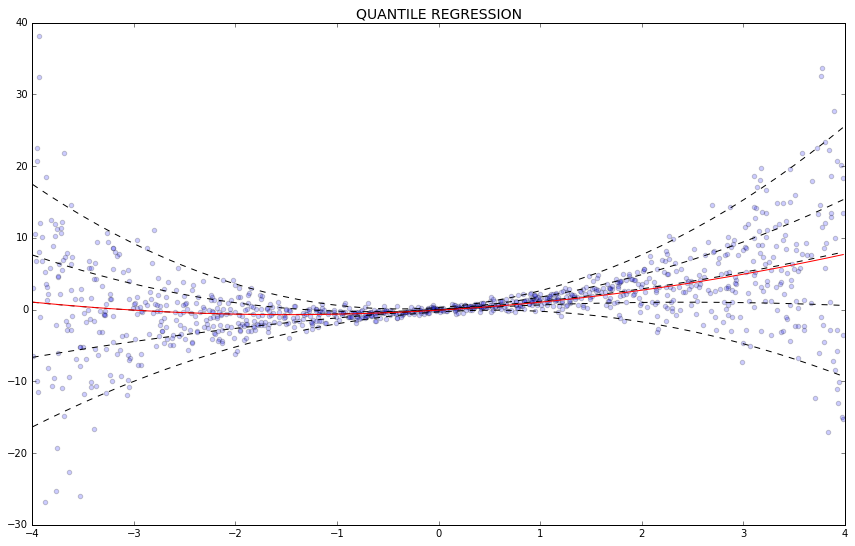This post is to demo the use of quantile regression analysis. As an example, we'll use a dataset where it is almost impossible to learn anything about the data using standard linear regression techniques. These methods were developed during a few weeks of work I recently completed for the Bridger-Teton Avalanche Center. Below is the code for 1st, 2nd, and 3rd-order polynomial linear regression, confidence and prediction intervals, and quantile regression.

Thanks to Josef Perktold at StatsModels for assistance with the quantile regression code, and providing the creative "heteroscedastic" dataset that we will analyze. (you'll have to look that word up on your own....)

First, let's generate some random data, and attempt to do some standard linear regression. We'll treat this as a correlation analysis, and call the x data 'predictor' and the y data 'response'.

In :
%matplotlib inline

In :
import numpy as np
import pandas as pd
import matplotlib.pyplot as plt
import statsmodels.formula.api as smf

np.random.seed(654123)

# generate a random dataset with heteroscedasticity
nobs = 1000
x = np.random.uniform(-4, 4, nobs)
y = x + 0.25 * x**2 + 0.1 * np.exp(1 + np.abs(x)) * np.random.randn(nobs)

df = pd.DataFrame({'predictor': x, 'response': y})

x1 = pd.DataFrame({'predictor': np.linspace(df.predictor.min(), df.predictor.max(), nobs)})

poly_1 = smf.ols(formula='response ~ 1 + predictor', data=df).fit()
poly_2 = smf.ols(formula='response ~ 1 + predictor + I(predictor ** 2.0)', data=df).fit()
poly_3 = smf.ols(formula='response ~ 1 + predictor + I(predictor ** 2.0) + I(predictor ** 3.0)', data=df).fit()

plt.figure(figsize=(9 * 1.618, 9))
plt.plot(x1.predictor, poly_1.predict(x1), 'r-',
label='1st order poly fit, $R^2$=%.2f' % poly_2.rsquared)
plt.plot(x1.predictor, poly_2.predict(x1), 'b-',
label='2nd order poly fit, $R^2$=%.2f' % poly_2.rsquared)
plt.plot(x1.predictor, poly_3.predict(x1), 'g-',
label='3rd order poly fit, $R^2$=%.2f' % poly_2.rsquared)

plt.plot(x, y, 'o', alpha=0.2)
plt.legend(loc="upper center", fontsize=14)

Out:With this particular dataset we learn almost nothing about the variability of the data from the linear regression models. Let's proceed with the 2nd order polynomial model, and have a look at confidence and prediction intervals. We'll also use the very nicely-formatted summary table from StatsModels to evaluate the polynomial fit.

In :
# CI and PI for 2nd order poly:

from statsmodels.stats.outliers_influence import summary_table
from statsmodels.sandbox.regression.predstd import wls_prediction_std

plt.figure(figsize=(9 * 1.618, 9))
plt.plot(x, y, 'o', alpha=0.2, label='')

plt.plot(x1.predictor, poly_2.predict(x1), 'k-', label='2nd order poly fit')
print poly_2.summary()

prstd, iv_l, iv_u = wls_prediction_std(poly_2)

st, data, ss2 = summary_table(poly_2, alpha=0.05)

fittedvalues = data[:,2]
predict_mean_se  = data[:,3]
predict_mean_ci_low, predict_mean_ci_upp = data[:,4:6].T
predict_ci_low, predict_ci_upp = data[:,6:8].T

# check we got the right things
#print np.max(np.abs(poly_2.fittedvalues - fittedvalues))
#print np.max(np.abs(iv_l - predict_ci_low))
#print np.max(np.abs(iv_u - predict_ci_upp))

data_intervals = {'predictor': x, 'predict_low': predict_ci_low, 'predict_upp': predict_ci_upp,
'conf_low': predict_mean_ci_low, 'conf_high': predict_mean_ci_upp}
df_intervals = pd.DataFrame(data=data_intervals)

df_intervals_sort = df_intervals.sort_values(by='predictor')

#embed()

plt.plot(df_intervals_sort.predictor, df_intervals_sort.predict_low,
color='r', linestyle='--', linewidth=2, label='95% prediction interval')
plt.plot(df_intervals_sort.predictor, df_intervals_sort.predict_upp,
color='r', linestyle='--', linewidth=2, label='')
plt.plot(df_intervals_sort.predictor, df_intervals_sort.conf_low,
color='c', linestyle='--', linewidth=2, label='95% confidence interval')
plt.plot(df_intervals_sort.predictor, df_intervals_sort.conf_high,
color='c', linestyle='--', linewidth=2, label='')

plt.legend(fontsize=14)

                            OLS Regression Results
==============================================================================
Dep. Variable:               response   R-squared:                       0.161
Model:                            OLS   Adj. R-squared:                  0.160
Method:                 Least Squares   F-statistic:                     95.99
Date:                Sat, 19 Mar 2016   Prob (F-statistic):           7.48e-39
Time:                        11:25:06   Log-Likelihood:                -3096.3
No. Observations:                1000   AIC:                             6199.
Df Residuals:                     997   BIC:                             6213.
Df Model:                           2
Covariance Type:            nonrobust
=======================================================================================
coef    std err          t      P>|t|      [95.0% Conf. Int.]
---------------------------------------------------------------------------------------
Intercept              -0.0845      0.257     -0.329      0.742        -0.588     0.419
predictor               0.8345      0.072     11.514      0.000         0.692     0.977
I(predictor ** 2.0)     0.2791      0.035      7.917      0.000         0.210     0.348
==============================================================================
Omnibus:                      177.059   Durbin-Watson:                   2.041
Prob(Omnibus):                  0.000   Jarque-Bera (JB):             3328.418
Skew:                           0.124   Prob(JB):                         0.00
Kurtosis:                      11.934   Cond. No.                         11.2
==============================================================================

Warnings:
 Standard Errors assume that the covariance matrix of the errors is correctly specified.

Out:The relatively tight confidence interval shows that the 2nd order polynomial model is accurately representing the mean of the response variable for any given predictor value. With the prediction interval, we can see that there is high variability somewhere in the model, such that a single new observation of the response variable for any given predictor value can deviate approximately +/-10 units from the model line (with 95% confidence).

However, we have still learned nothing about the distribution of the data. Linear regression techniques are not appropriate to analyze this dataset.

Now we'll apply quantile regression:

In :
mod = smf.quantreg('response ~ predictor + I(predictor ** 2.0)', df)

# Quantile regression for 5 quantiles

quantiles = [.05, .25, .50, .75, .95]

# get all result instances in a list
res_all = [mod.fit(q=q) for q in quantiles]

res_ols = smf.ols('response ~ predictor + I(predictor ** 2.0)', df).fit()

plt.figure(figsize=(9 * 1.618, 9))

# create x for prediction
x_p = np.linspace(df.predictor.min(), df.predictor.max(), 50)
df_p = pd.DataFrame({'predictor': x_p})

for qm, res in zip(quantiles, res_all):
# get prediction for the model and plot
# here we use a dict which works the same way as the df in ols
plt.plot(x_p, res.predict({'predictor': x_p}), linestyle='--', lw=1, color='k')

y_ols_predicted = res_ols.predict(df_p)
plt.plot(x_p, y_ols_predicted, color='red', label='OLS')
plt.scatter(df.predictor, df.response, alpha=.2)
plt.xlim((-4, 4))
plt.ylim((-30, 40))
plt.title('QUANTILE REGRESSION', fontsize=14)

Out:The red line is the 2nd order polynomial model, and the black dashed lines represent the 5th, 25th, 50th, 75th, and 95th quantiles of the regression. We can see that the linear regression model and the 50th percentile are very similar (the mean and the median are similar). We have now also shown the distribution of the data. In this particular case, the quantiles are symmetric. If the quantiles showed different slopes throughout the distribution of data (non-symmetric), we could potentially learn something about different processes that affect the response variable for different ranges of the data.

In general quantile regression is useful for:

• Examining relationships throughout the distribution of the response variable, not just the mean response.

• Calculation of various prediction intervals for non-parametric (non-normal) data.